# RS Aggarwal Solutions for Class 8 Maths Chapter 19 - Three-dimensional Figures Exercise 19A

To excel in board exams, students can download the RS Aggarwal Solutions for the Exercise 19A of Class 8 Maths Chapter 19, Three-Dimensional Figures from the link available here. BYJU’S expert team has designed solutions efficiently that helps students solve problems in the most efficient ways possible. The solutions prepared are according to the RS Aggarwal Solutions guidelines to understand every concept. By practising the RS Aggarwal Solutions for Class 8, students will be able to grasp the concepts correctly. Hence, students whose aim is to score high in Maths examinations are advised to go through RS Aggarwal Solutions for Class 8.

## Download PDF of RS Aggarwal Solutions for Class 8 Maths Chapter 19 – Three-Dimensional Figures – Exercise 19A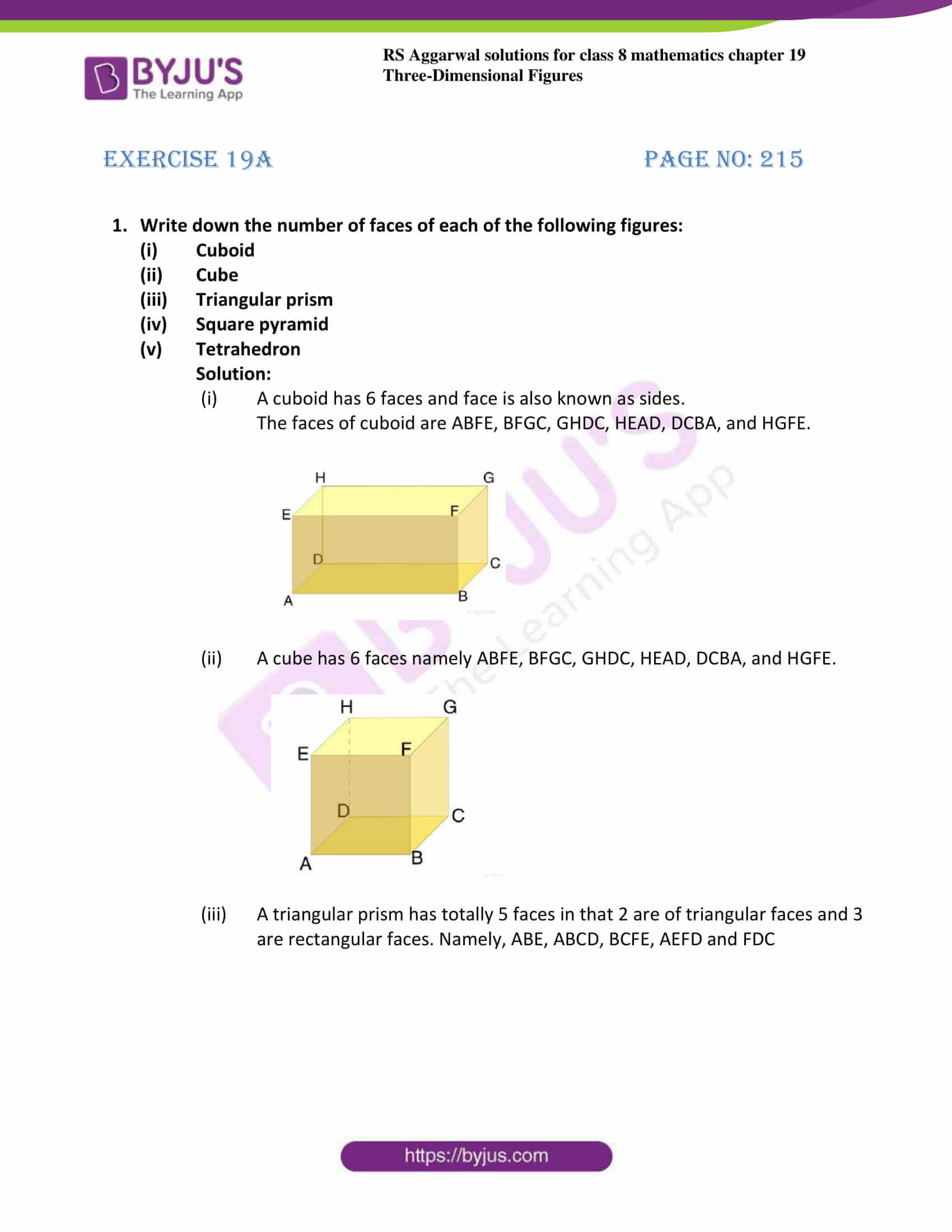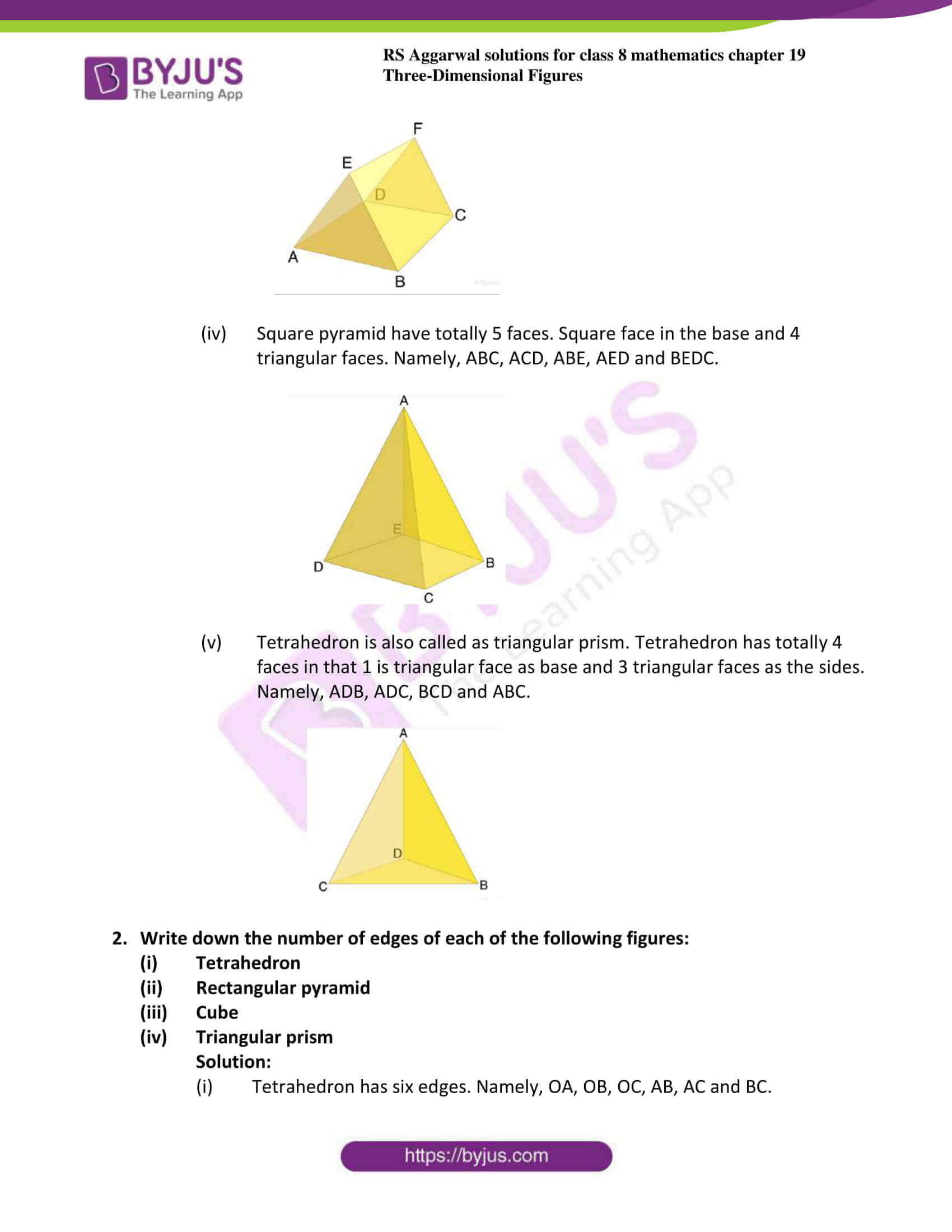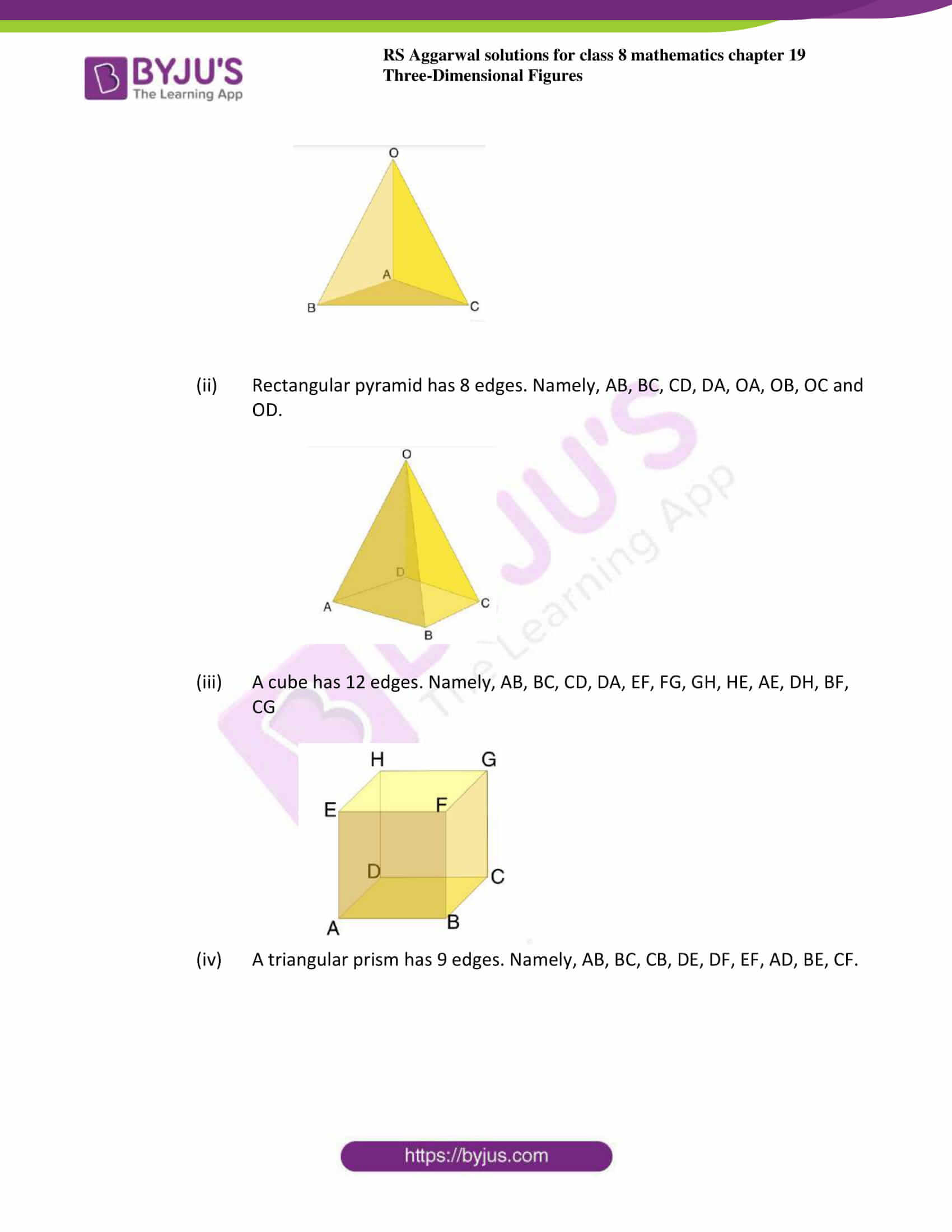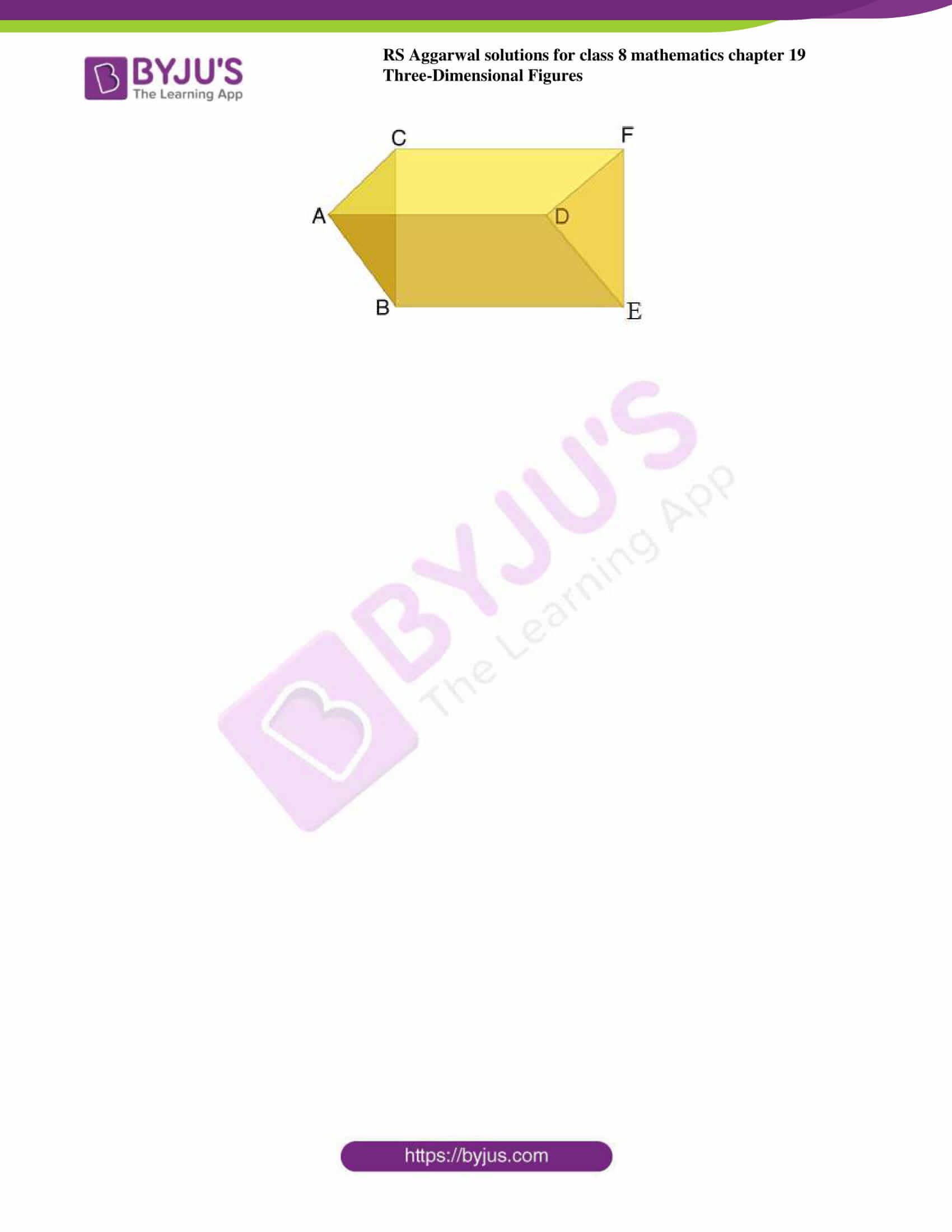### Access answers to Maths RS Aggarwal Solutions for Class 8 Chapter 19 – Three-Dimensional Figures Exercise 19A

1. Write down the number of faces of each of the following figures:

1. Cuboid
2. Cube
3. Triangular prism
4. Square pyramid
5. Tetrahedron

Solution:

1. A cuboid has 6 faces and face is also known as sides.The faces of cuboid are ABFE, BFGC, GHDC, HEAD, DCBA, and HGFE.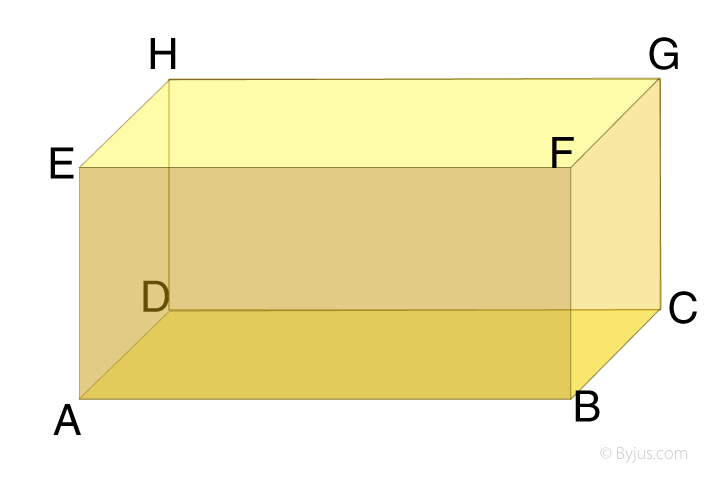2. A cube has 6 faces namely ABFE, BFGC, GHDC, HEAD, DCBA, and HGFE.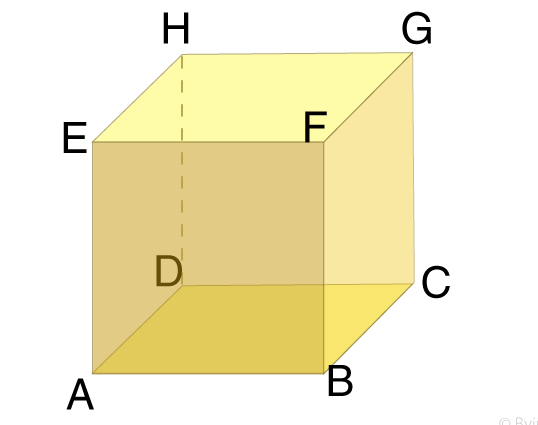3. A triangular prism has totally 5 faces in that 2 are of triangular faces and 3 are rectangular faces. Namely, ABE, ABCD, BCFE, AEFD and FDC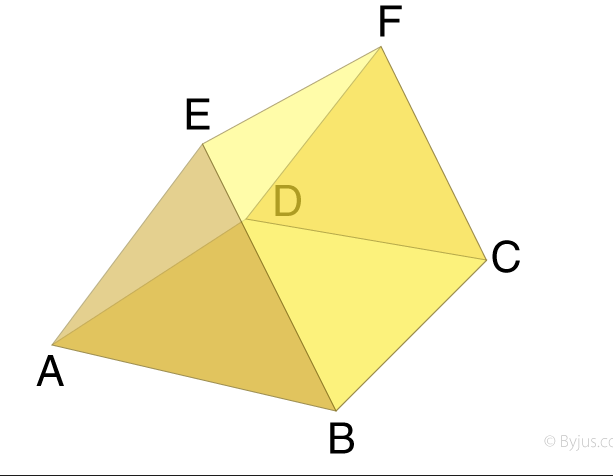4. Square pyramid have totally 5 faces. Square face in the base and 4 triangular faces. Namely, ABC, ACD, ABE, AED and BEDC.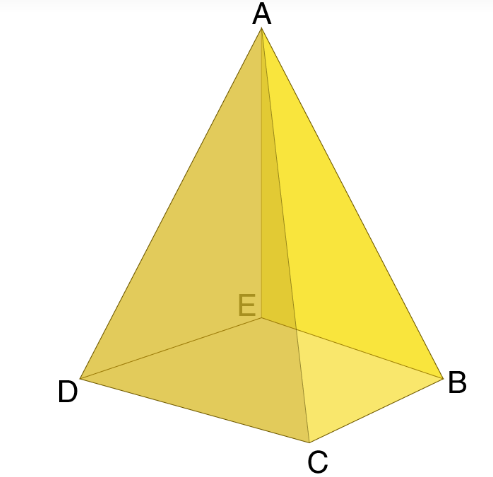v. Tetrahedron is also called as triangular prism. Tetrahedron have totally 4 faces in that 1 is triangular face as base and 3 triangular faces as the sides. Namely, ADB, ADC, BCD and ABC.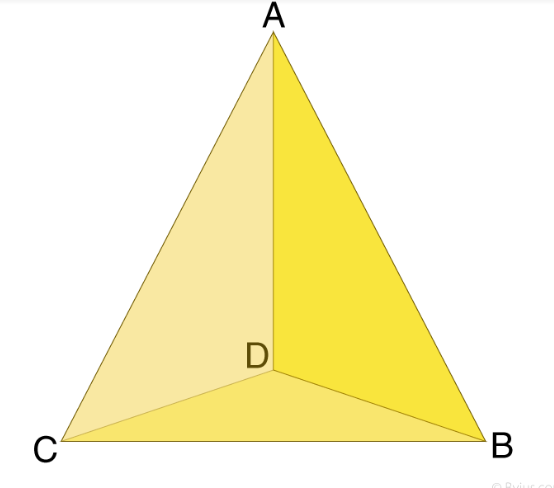2. Write down the number of edges of each of the following figures:

1. Tetrahedron
2. Rectangular pyramid
3. Cube
4. Triangular prism

Solution:

1. Tetrahedron has six edges. Namely, OA, OB, OC, AB, AC and BC.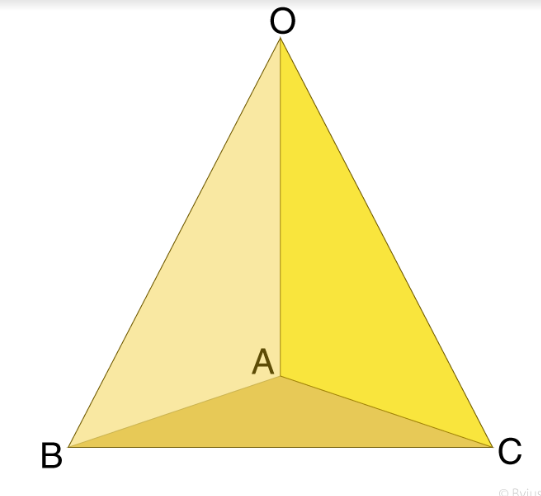2. Rectangular pyramid has 8 edges. Namely, AB, BC, CD, DA, OA, OB, OC and OD.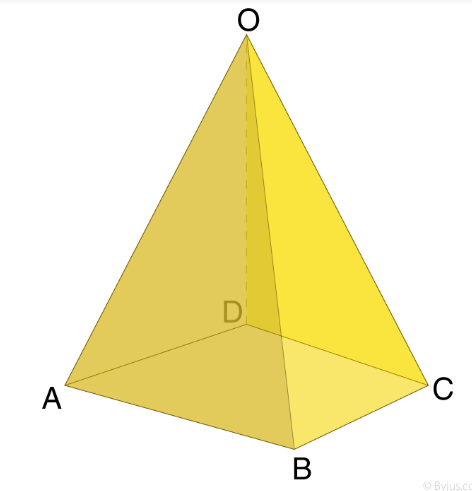3. A cube has 12 edges. Namely, AB, BC, CD, DA, EF, FG, GH, HE, AE, DH, BF, CG.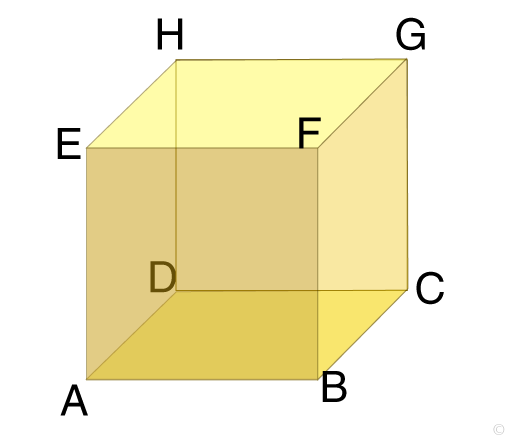4. A triangular prism has 9 edges. Namely, AB, BC, CB DE, DF, EF, AD, BE, CF.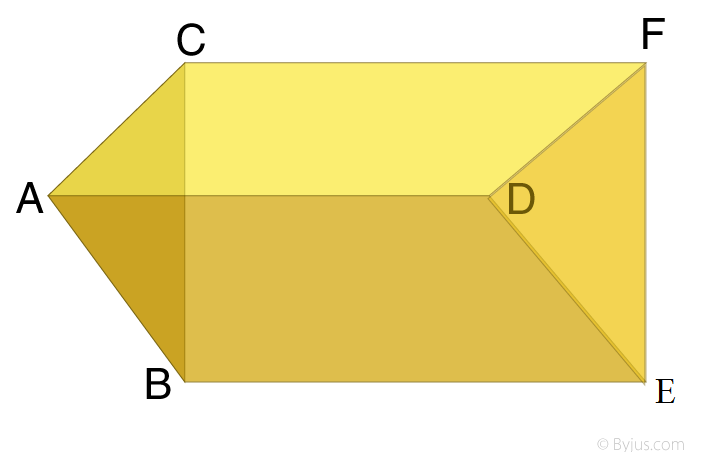### Access another exercise of RS Aggarwal Solutions for Class 8 Maths Chapter 19 – Three-Dimensional Figures

Exercise 19B Solutions 5 questions

## RS Aggarwal Solutions for Class 8 Maths Chapter 19 – Three-Dimensional Figures Exercise 19A

Exercise 19A of RS Aggarwal Solutions for Chapter 19, Three-dimensional Figures deals with the basic concepts related to three-dimensional figures. We can say that this exercise mainly deals with the faces, vertices and edges of Three-Dimensional Figures that the students have learnt in this Chapter.

The RS Aggarwal Solutions help the students to practise and learn each and every concept as it provides answers to all questions asked in the textbook. Those who aim to score high in Class 8 Maths are advised to practise all the questions present in RS Aggarwal as many times as possible.